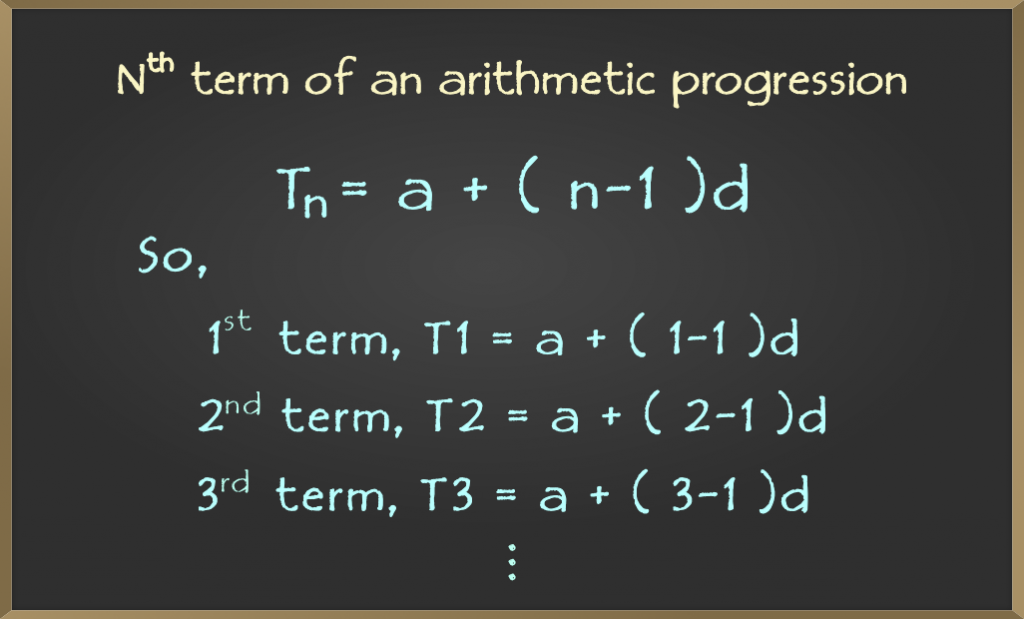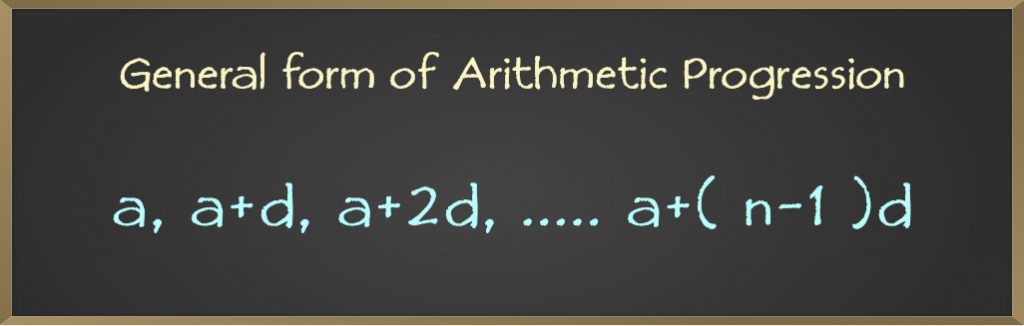Related Articles

# Introduction to Arithmetic Progressions | Class 10 Maths

• Difficulty Level : Medium
• Last Updated : 09 Nov, 2020

A progression is a sequence or series of numbers in which they are arranged in a particular order such that the relation between the consecutive terms of series or sequence is always constant. In a progression, it is possible to obtain the nth term of the series.

In mathematics, there are 3 types of progressions:

1. Arithmetic Progression(AP)
2. Geometric Progression(GP)
3. Harmonic Progression(HP)

Arithmetic Progression (AP) also known as Arithmetic Sequence is a sequence or series of numbers such that the common difference between two consecutive numbers in the series is constant.

For example:

Series 1: 1,3,5,7,9,11….

In this series, the common difference between any two consecutive numbers is always 2.

Series 2: 28,25,22,19,16,13….

In this series, the common difference between any two consecutive numbers is strictly -3.

### Terminology and Representation

• Common difference, d = a2 – a1 = a3 – a2 = ……. = an – an – 1
• an = nth term of Arithmetic Progression
• Sn = Sum of first n elements in the series

### General Form of an AP

If a is taken as the first term and d is taken as the common difference, then the Nth term of the AP will be given by the formula:So by computing the n terms of an AP with the upgiven formula, the general form of the AP is as follows:Example: Find the 35th term of the series 5,11,17,23…..

Solution:

In the given series,

a = 5, d = a2 – a1 = 11 – 5 = 6, n = 35

We have to find out the 35th term, hence, apply the formulae,

an = a + (n – 1)d

an = 5 + (35 – 1) x 6

an = 5 + 34 x 6

an = 209

Hence 209 is the 35th term.

### Sum of n Terms of Arithmetic Progression

The formula for the arithmetic progression sum is,

Sn = (n/2)[2a + (n − 1) × d]

### Derivation of the Formula

Let ‘l’ denote the nth term of the series and Sn be the sun of first n terms of AP a, (a+d), (a+2d), …., a+(n-1)d then,

Sn = a1 + a2 + a3 + ….an-1 + an

Sn = a + (a + d) + (a + 2d) + …….. + (l – 2d) + (l – d) + l                      …(1)

Writing the series in reverse order, we get,

Sn = l + (l – d) + (l – 2d) + …….. + (a + 2d) + (a + d) + a                      …(2)

2Sn = (a + l) + (a + l) + (a + l) + …….. + (a + l) + (a + l) + (a + l)

2Sn = n(a + l)

Sn = (n/2)(a + l)             …(3)

Hence, the formulae for finding the sum of a series is,

Sn = (n/2)(a + l)

where,

a is the first term

l is the last term of the series and

n is the number of terms in the series

Replacing the last term l by the nth term in equation 3 we get,

nth term = a + (n – 1)d

Sn = (n/2)(a + a + (n – 1)d)

Sn = (n/2)(2a + (n – 1) x d)

Note: The consecutive terms in an Arithmetic Progression can also be represented as,

…….., a-3d , a-2d, a-d, a, a+d, a+2d, a+3d, ……..

### Sample Problems on Arithmetic Progressions

Problem 1. Find the sum of the first 35 terms of series 5,11,17,23…..

Solution:

In the given series,

a = 5, d = a2 – a1 = 11 – 5 = 6, n = 35

Sn = (n/2)(2a + (n – 1) x d)

Sn = (35/2)(2 x 5 + (35 – 1) x 6)

Sn = (35/2)(10 + 34 x 6)

Sn = (35/2)(10 + 204)

Sn = 35 x 214/2

Sn = 3745

Problem 2. Find the sum of the series when the first term of the series is 5 and the last of the series is 209 and the number of terms in the series is 35.

Solution:

In the given series,

a = 5, l = 209, n = 35

Sn = (n/2)(a + l)

Sn = (35/2)(5 + 209)

Sn = 35 x 214/2

Sn = 3745

Problem 3. 21 Rupees is divided among three brothers where the three parts of money are in AP and the sum of their squares is 155. Find the largest amount.

Solution:

Let the three parts of money be (a-d), a, (a+d), as thee distributed amount is in AP

Given that

(a – d) + a + (a + d) = 21

Therefore,

3a = 21

a = 7

Again, (a – d)2 + a2 + (a + d)2 = 155

a2 + d2 – 2ad + a2 + a2 + d2 + 2ad = 155

3a2 + 2d2 = 155

Putting the value of ‘a’ we get,

3(7)2 + 2d2 = 155

2d2 = 155 – 147

d2 = 4

d = ±2

The three parts of distributed money are:

a + d = 7 + 2 = 9

a = 7

a – d = 7 – 2 = 5

Hence, the largest part is Rupees 9

Attention reader! Don’t stop learning now. Participate in the Scholorship Test for First-Step-to-DSA Course for Class 9 to 12 students.

My Personal Notes arrow_drop_up